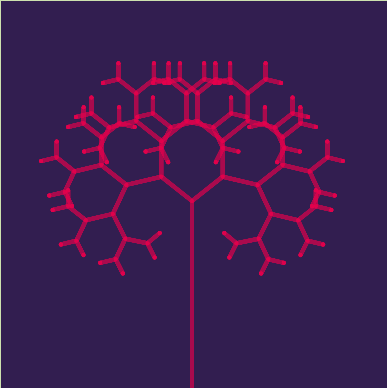Skip to content

# Demonstrandum/BasicCanvas

A friendlier way to interact with the canvas; simple library for canvas manipulation.Fetching latest commit…
Cannot retrieve the latest commit at this time.
Type Name Latest commit message Commit time
Failed to load latest commit information.docsexamplelib.esdoc.json.gitignore.nowignoreLICENSEREADME.mdindex.htmlnow.jsonpackage.jsonserver.sh

# BasicCanvas

A friendlier way interact with the canvas.

## Usage

jsdelivr CDN (use this to import):

• Canvas
``````https://cdn.jsdelivr.net/gh/Demonstrandum/BasicCanvas@v1.1.1/lib/BasicCanvas.js
``````
• Shapes
``````https://cdn.jsdelivr.net/gh/Demonstrandum/BasicCanvas@v1.1.1/lib/BasicShapes.js
``````
• DOM
``````https://cdn.jsdelivr.net/gh/Demonstrandum/BasicCanvas@v1.1.1/lib/BasicDOM.js
``````

### Docs

Auto-generated documentation: canvas.knutsen.co/docs/

TODO: Instructions on usage, for now look at the example files (and/or source files), still a small project.

## Run Examples

Example hosted with ▲now: canvas.knutsen.co

To run unlisted examples, or run specific examples by a specifc URL, simply go to:

First clone:

```git clone https://github.com/Demonstrandum/BasicCanvas.git
cd BasicCanvas```

then run with:

`./server.sh`

And go to http://localhost:8000/example/ (for an example file, see the index.html code to switch example).

## Quick ExamplesSee Animation.

```import * as BC from '../lib/BasicCanvas.js';
import {line} from '../lib/BasicShapes.js';

use(BC);

const sketch = canvas_id('sketch');
sketch.dimensions(400, 400);
sketch.translate(sketch.width / 2, sketch.height / 2);

sketch.fill = RGB(50, 30, 80);
sketch.stroke = HSL(340, 100, 45, 170);
sketch.stroke_weight = 4;
sketch.stroke_cap = 'round';

const branch = (previous, angle, depth, inc, first = true) => {
if (depth < 0) return;

let next = previous;
if (!first) {
next = Polar(Math.sqrt(depth) * 16, -angle, previous);
sketch.render(line(next, previous));
}

branch(next, angle + inc, depth - 1, inc, false);
branch(next, angle - inc, depth - 1, inc, false);
};

const tree = P(0, 10);
sketch.loop(frame => {
sketch.background();
sketch.render(line(P(0, 200), tree));
branch(tree, Math.PI / 2, 7, 0.6 + Math.sin(frame / 20) / 3);
});```

Drawing a simple sinusoidal progressive wave:

```import * as BC from 'https://cdn.jsdelivr.net/gh/Demonstrandum/BasicCanvas@v1.1.1/lib/BasicCanvas.js';
// If running this locally, you need a server running for `import`s to work, (for now).

use(BC)  // To avoid having to write `BC.` all the time.
// (Be ware of collisions)

const sketch = canvas_id('sketch'); // Gets canvas with id="sketch".
sketch.dimensions(400, 400); // width x height, size of the canvas.

sketch.stroke = RGB(0); // Same as BC.RGBA(0, 0, 0, 255).
sketch.stroke_weight = 8; // 8px wide.
sketch.stroke_cap = 'round';

const BG = RGB(255, 255, 110);
sketch.loop(frame => {  // `frame` is an integer, starts at 0 and increments for every frame drawn.
sketch.background(BG); // Redraw background each frame.

sketch.render('sine', shape => { // Create new shape, `shape(name, construction of shape callback)`
for (let x = 0; x < 3 * Math.PI; x += 0.2) { // Draw sine curve for this frame, next frame will be different
shape.vertex(BC.Point(32 * x + 50, 32 * Math.sin(x + frame / 10) + 200));
}
});
});```

Make sure the relative path to the BasicCanvas.js file is correct.

If the above file is called something like `sine_wave.js` then the `index.html` file (in the same folder) should look something like:

```<!DOCTYPE html>
<html lang="en" dir="ltr">
<head>
<meta charset="utf-8">
<title>Simple Sinusoidal Wave</title>
</head>
<body>
<canvas id="sketch"></canvas>
<script src="sine_wave.js" type="module" charset="utf-8"></script>
</body>
</html>```

Or, you could use the `your_example.js` file found in the example/ folder of the repo.

## Try Yourself

Check out the CodePen: https://codepen.io/wernstrom/project/editor/DKzVaY Explore the library by making small modifications to the CodePen and/or rewriting it to do something new.

You can’t perform that action at this time.